# km.pmf

## Introduction

The km.pmf function is part of the conf package. The function calculates the probability mass function for the support values of Kaplan and Meier’s product–limit estimator1. The Kaplan-Meier product-limit estimator (KMPLE) is used to estimate the survivor function for a data set of positive values in the presence of right censoring. The km.pmf function generates the probability mass function for the support values of the KMPLE for a particular sample size n, probability of observing a failure h at the time of interest expressed as the cumulative probability perc associated with $$X = \min(T, C)$$, where $$T$$ is the failure time and $$C$$ is the censoring time under a random-censoring scheme.

## Installation Instructions

The km.pmf function is accessible following installation of the conf package:

install.packages("conf")
library(conf)

## Details

The KMPLE is a nonparametric estimate of the survival function from a data set of lifetimes that includes right-censored observations and is used in a variety of application areas. For simplicity, we will refer to the object of interest generically as the item and the event of interest as the failure.

Let $$n$$ denote the number of items on test. The KMPLE of the survival function $$S(t)$$ is given by $\hat{S}(t) = \prod\limits_{i:t_i \leq t}\left( 1 - \frac{d_i}{n_i}\right),$ for $$t \ge 0$$, where $$t_1, \, t_2, \, \ldots, \, t_k$$ are the times when at least one failure is observed ($$k$$ is an integer between 1 and $$n$$, which is the number of distinct failure times in the data set), $$d_1, \, d_2, \, \ldots, \, d_k$$ are the number of failures observed at times $$t_1, \, t_2, \, \ldots, \, t_k$$, and $$n_1, \, n_2, \, \ldots, \, n_k$$ are the number of items at risk just prior to times $$t_1, \, t_2, \, \ldots, \, t_k$$. It is common practice to have the KMPLE “cut off” after the largest time recorded if it corresponds to a right-censored observation2. The KMPLE drops to zero after the largest time recorded if it is a failure; the KMPLE is undefined (NA), however, after the largest time recorded if it is a right-censored observation.

The support values (calculated in km.support) are calculated from $$\hat{S}(t)$$ at any $$t \ge 0$$ for all possible outcomes of an experiment with $$n$$ items on test.

The function km.pmf calculates the probability for each support value and produces a plot of the probabilities. The probability of NA, the event that the last time recorded is a right-censored observation, is also calculated and plotted at the arbitrary position of s = 1.1.

### Required Arguments

n sample size

h probability of observing a failure; that is, P(X = T)

perc cumulative probability associated with X = (T, C)

### Optional Arguments

plot option to plot the probability mass function (default is TRUE)

sep option to show the breakdown of the probability for each support value (see function km.outcomes for details on the breakdown) (default is TRUE)

xfrac option to label support values on the x-axis as exact fractions (default is TRUE)

cex.lollipop size of the dots atop the spikes

## Examples

The following section provides various examples for the usage of km.pmf.

### Example 1

Qin et al.3 derived the probability mass function of the KMPLE for one particular setting where there are $$n = 3$$ items on test, the failure times $$T_1,T_2$$ and $$T_3$$ and the censoring times $$C_1,C_2$$ and $$C_3$$ both follow an exponential(1) distribution. The fixed time of interest is $$t_0 = -\ln(1/2)/2$$, which is the median of $$X = \min(T, C)$$, where $$T$$ is the failure time and $$C$$ is the censoring time under a random-censoring scheme. Therefore, perc = 0.5.

In this case, since failure and censoring times have the same exponential distribution, they are equally likely to occur; that is, h = 1/2.

For this example, km.pmf is called with the arguments n = 3, h = 0.5, and perc = 0.5. The optional defaults are used for this example. Two columns of output are produced: the support values and their probabilities. In addition, by default, a plot of the probability mass function is created with hash marks on the point mass lines to show the breakdown of the probability for each of the support values for the different possible outcomes (see function km.outcomes for details).

library(conf)
#  display the probability mass function

km.pmf(n=3, h = 1/2, perc = 0.5)
#> Additional delay due to printing hash marks.
#>           S       P
#> 1 0.0000000 0.06250
#> 2 0.3333333 0.09375
#> 3 0.5000000 0.09375
#> 4 0.6666667 0.28125
#> 5 1.0000000 0.40625
#> 6        NA 0.06250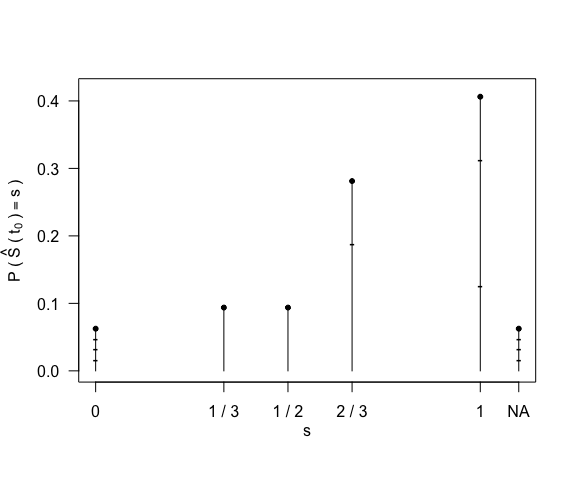### Example 2

In other experiments, it may be more reasonable to expect that we have a higher or lower chance of censoring. In this example we will start with a higher rate of right-censoring, so a lower chance that we will observe a failure. Set h = 1/3. We may also want to look at different values of $$t_0$$. Here we will choose the 75th percentile of $$X = \min(T, C)$$; that is, perc = 0.75. In addition, we will remove the hash marks, use decimals on the $$x$$-axis, and increase the size of the dot on top of the point masses.

#  display the probability mass function with lower failure rate (higher censoring rate)

km.pmf(n=3, h = 1/3, perc = 0.75, sep = FALSE, xfrac = FALSE, cex.lollipop = 2)
#>           S        P
#> 1 0.0000000 0.140625
#> 2 0.3333333 0.046875
#> 3 0.5000000 0.093750
#> 4 0.6666667 0.140625
#> 5 1.0000000 0.296875
#> 6        NA 0.281250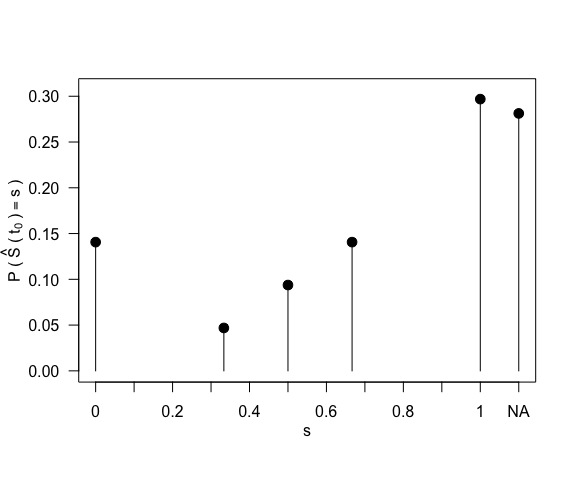For comparison purposes, the following plot and probability mass function have an earlier time of interest (perc = 0.35) so it is less likely that all of the observations have occurred making the probability of survival less likely to be 0 or NA, which occur at the end of the experiment. We can also see with the higher rate of censoring that early in the experiment there is a high probability of survival equal to 1. This point mass represents all possible censored items before a failure at the 35th percentile of $$X = \min(T, C)$$.

#  display the probability mass function with an earlier time of interest

km.pmf(n=3, h = 1/3, perc = 0.35, sep = FALSE, xfrac = FALSE, cex.lollipop = 2)
#>           S          P
#> 1 0.0000000 0.01429167
#> 2 0.3333333 0.02654167
#> 3 0.5000000 0.05308333
#> 4 0.6666667 0.20095833
#> 5 1.0000000 0.67654167
#> 6        NA 0.02858333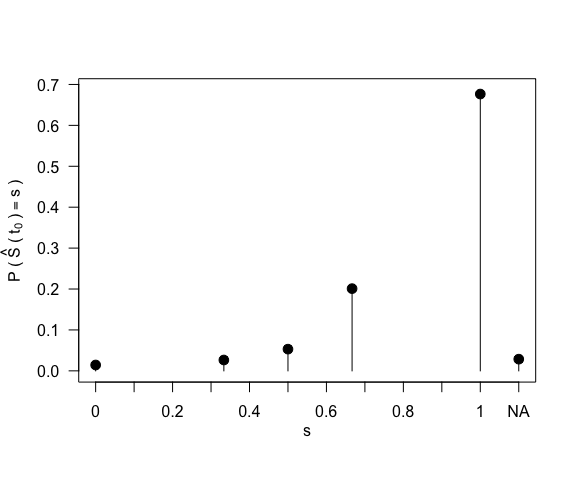In addition, instead of examining a different time of interest, we can look at a higher failure rate. For the following plot and probability mass function, we set h = 2/3. We return to the 75th percentile (perc = 0.75). With a lower probability of censoring, we have smaller probabilities at 1 and NA than in the first plot of this example. There is lower probability of 1 because we are more likely to observe at least one failure, and there is lower probability of NA (and higher probability of 0) because we are more likely (with probability 2/3) to observe a failure for the last item on test.

#  display the probability mass function with a higher probability of failure (lower censoring rate)

km.pmf(n=3, h = 2/3, perc = 0.75, sep = FALSE, xfrac = FALSE, cex.lollipop = 2)
#>           S        P
#> 1 0.0000000 0.281250
#> 2 0.3333333 0.187500
#> 3 0.5000000 0.093750
#> 4 0.6666667 0.187500
#> 5 1.0000000 0.109375
#> 6        NA 0.140625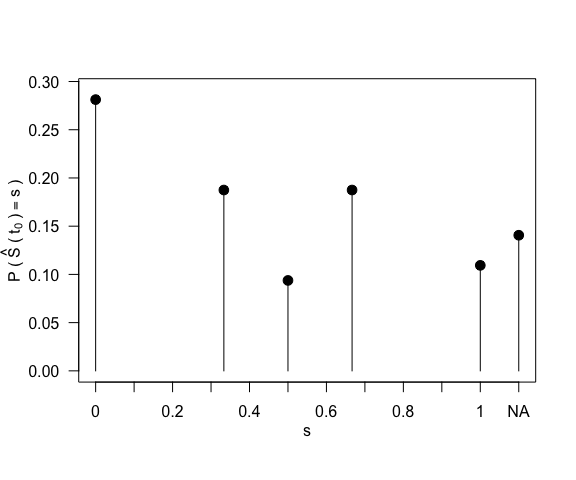### Example 3

Since all possible outcomes and probabilities are calculated, large sample size n may affect the speed of the function. Due to CPU and memory limitations, n is limited to values from 1 to 23. For a larger sample size n, it is recommended to set sep = FALSE to remove the hash marks to reduce the delay in rendering the plot, and xfrac = FALSE (removing the exact fractions from the $$y$$-axis) and cex.lollipop = 0.01 (making tiny dots on top of the point masses) for a better visual effect.

#  display the probability mass function with a sample size of 8
km.pmf(8, 1/2, 0.75, sep = FALSE, xfrac = FALSE, cex.lollipop = 0.01)
#>            S           P
#> 1  0.0000000 0.050056458
#> 2  0.1250000 0.002085686
#> 3  0.1428571 0.002085686
#> 4  0.1458333 0.002085686
#> 5  0.1500000 0.002085686
#> 6  0.1562500 0.002085686
#> 7  0.1666667 0.004171371
#> 8  0.1714286 0.002085686
#> 9  0.1750000 0.002085686
#> 10 0.1785714 0.002085686
#> 11 0.1822917 0.002085686
#> 12 0.1875000 0.004171371
#> 13 0.1904762 0.002085686
#> 14 0.1944444 0.002085686
#> 15 0.2000000 0.004171371
#> 16 0.2083333 0.004171371
#> 17 0.2142857 0.004171371
#> 18 0.2187500 0.004171371
#> 19 0.2222222 0.002085686
#> 20 0.2250000 0.002085686
#> 21 0.2285714 0.002085686
#> 22 0.2333333 0.002085686
#> 23 0.2343750 0.002085686
#> 24 0.2380952 0.002085686
#> 25 0.2430556 0.002085686
#> 26 0.2500000 0.015295029
#> 27 0.2571429 0.002085686
#> 28 0.2625000 0.002085686
#> 29 0.2666667 0.002085686
#> 30 0.2678571 0.002085686
#> 31 0.2734375 0.002085686
#> 32 0.2777778 0.002085686
#> 33 0.2812500 0.002085686
#> 34 0.2857143 0.011123657
#> 35 0.2916667 0.011123657
#> 36 0.3000000 0.011123657
#> 37 0.3125000 0.011123657
#> 38 0.3214286 0.002085686
#> 39 0.3281250 0.002085686
#> 40 0.3333333 0.018075943
#> 41 0.3428571 0.009037971
#> 42 0.3500000 0.009037971
#> 43 0.3571429 0.009037971
#> 44 0.3645833 0.009037971
#> 45 0.3750000 0.024564743
#> 46 0.3809524 0.006952286
#> 47 0.3888889 0.006952286
#> 48 0.4000000 0.015990257
#> 49 0.4166667 0.015990257
#> 50 0.4285714 0.022479057
#> 51 0.4375000 0.022479057
#> 52 0.4444444 0.006952286
#> 53 0.4500000 0.013441086
#> 54 0.4571429 0.006952286
#> 55 0.4666667 0.006952286
#> 56 0.4687500 0.013441086
#> 57 0.4761905 0.006952286
#> 58 0.4861111 0.006952286
#> 59 0.5000000 0.048279762
#> 60 0.5142857 0.013441086
#> 61 0.5250000 0.013441086
#> 62 0.5333333 0.006952286
#> 63 0.5357143 0.013441086
#> 64 0.5468750 0.013441086
#> 65 0.5555556 0.006952286
#> 66 0.5625000 0.013441086
#> 67 0.5714286 0.025800705
#> 68 0.5833333 0.025800705
#> 69 0.6000000 0.032289505
#> 70 0.6250000 0.035173416
#> 71 0.6428571 0.013441086
#> 72 0.6562500 0.013441086
#> 73 0.6666667 0.025800705
#> 74 0.6857143 0.018848419
#> 75 0.7000000 0.018848419
#> 76 0.7142857 0.021732330
#> 77 0.7291667 0.021732330
#> 78 0.7500000 0.036134720
#> 79 0.8000000 0.018848419
#> 80 0.8333333 0.021732330
#> 81 0.8571429 0.022693634
#> 82 0.8750000 0.022876740
#> 83 1.0000000 0.022891998
#> 84        NA 0.050056458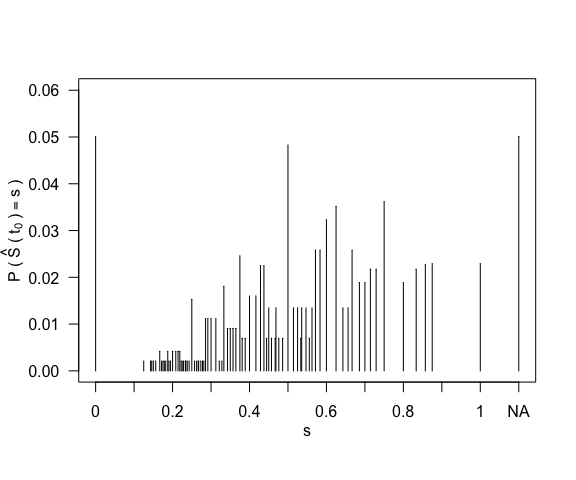## Package Notes

For more information on how the $$\hat{S}(t)$$ values are generated, please refer to the vignette titled km.support.

For more information on the hash marks generated on the plot, please refer to the vignette titled km.outcomes.

In addition, km.pmf calls the functions km.support and km.outcomes.

These functions and vignettes are both available via the link on the conf package webpage.

1. Kaplan, E. L., and Meier, P. (1958), “Nonparametric Estimation from Incomplete Observations,” Journal of the American Statistical Association, 53, 457–481.↩︎

2. Kalbfleisch, J. D., and Prentice, R. L. (2002), The Statistical Analysis of Failure Time Data (2nd ed.), Hoboken, NJ: Wiley.↩︎

3. Qin Y., Sasinowska H. D., Leemis L. M. (2023), “The Probability Mass Function of the Kaplan–Meier Product–Limit Estimator,” The American Statistician, 77 (1), 102–110.↩︎